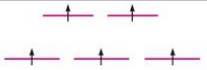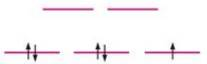# Match the crystal field diagrams given below with the following complex ions. Fe(CN) 6 3− Mn(H 2 O) 6 2+ (a) (b)### Chemistry: An Atoms First Approach

2nd Edition
Steven S. Zumdahl + 1 other
Publisher: Cengage Learning
ISBN: 9781305079243

#### Solutions

Chapter
Section### Chemistry: An Atoms First Approach

2nd Edition
Steven S. Zumdahl + 1 other
Publisher: Cengage Learning
ISBN: 9781305079243
Chapter 20, Problem 50E
Textbook Problem
1 views

## Match the crystal field diagrams given below with the following complex ions.Fe(CN)63−Mn(H2O)62+(a)(b)(a)

Interpretation Introduction

Interpretation: The given crystal field diagrams are to be matched with the correct complex ions.

Concept introduction: Weak field ligand that has small crystal field splitting energy gives high spin due to presence of maximum unpaired electrons in lower energy t2g orbitals and higher energy orbitals.

Strong field ligand that has large crystal field splitting energy gives low spin due to presence of maximum paired electrons in lower energy t2g orbitals.

To determine: If the given crystal field diagram corresponds to Fe(CN)63 ion or Mn(H2O)62+ ion.

### Explanation of Solution

Explanation

The given crystal field diagram corresponds to Mn(H2O)62+ ion.

The given crystal field diagram is,

Figure 1

The complex compound is Mn(H2O)62+ .

Oxidation state of weak ligand Water (H2O) is 0 .

It is assumed that oxidation state of metal ion Manganese (Mn) is x

(b)

Interpretation Introduction

Interpretation: The given crystal field diagrams are to be matched with the correct complex ions.

Concept introduction: Weak field ligand that has small crystal field splitting energy gives high spin due to presence of maximum unpaired electrons in lower energy t2g orbitals and higher energy orbitals.

Strong field ligand that has large crystal field splitting energy gives low spin due to presence of maximum paired electrons in lower energy t2g orbitals.

To determine: If the given crystal field diagram corresponds to Fe(CN)63 ion or Mn(H2O)62+ ion.

### Still sussing out bartleby?

Check out a sample textbook solution.

See a sample solution

#### The Solution to Your Study Problems

Bartleby provides explanations to thousands of textbook problems written by our experts, many with advanced degrees!

Get Started

Find more solutions based on key concepts
Name the following compounds. a Cu(NO2)3 b (NH4)3P c K2SO3 d Hg3N2

General Chemistry - Standalone book (MindTap Course List)

Trabecular bone readily gives up its minerals whenever blood calcium needs replenishing. T F

Nutrition: Concepts and Controversies - Standalone book (MindTap Course List)

Fill in the following table:

Chemistry: Principles and Reactions

What impact has recombinant DNA technology had on genetics and society?

Human Heredity: Principles and Issues (MindTap Course List)

Review. A student proposes to study the gravitational force by suspending two 100.0-kg spherical objects at the...

Physics for Scientists and Engineers, Technology Update (No access codes included)

What atom has only one proton? a. hydrogen b. an isotope c. a free radical d. a radioisotope

Biology: The Unity and Diversity of Life (MindTap Course List)